Get instant live expert help with Excel or Google Sheets“My Excelchat expert helped me in less than 20 minutes, saving me what would have been 5 hours of work!”

#### Post your problem and you’ll get expert help in seconds.

Your message must be at least 40 characters
Our professional experts are available now. Your privacy is guaranteed.
All articles AVERAGE Discover How to Calcuate an Exponential Moving Average in Excel

# Discover How to Calcuate an Exponential Moving Average in Excel

We can use the Data analysis option in excel to calculate an exponential moving average. An exponential moving average (EMA) places more emphasis on recent data points than a simple moving average which considers all observations within a period. The steps below will walk through the process.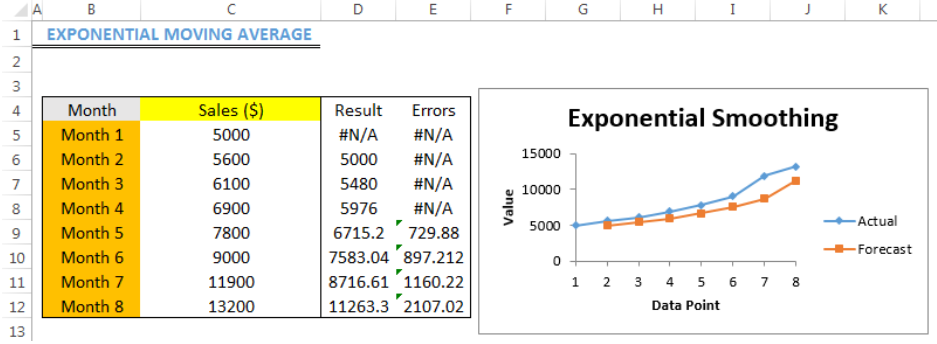Figure 1- How to Calculate an Exponential Moving Average in Excel

## Inserting Data Analysis Option

If you do not have the Data analysis option, follow these steps:

• Click on File, click on options, click on Add-Ins,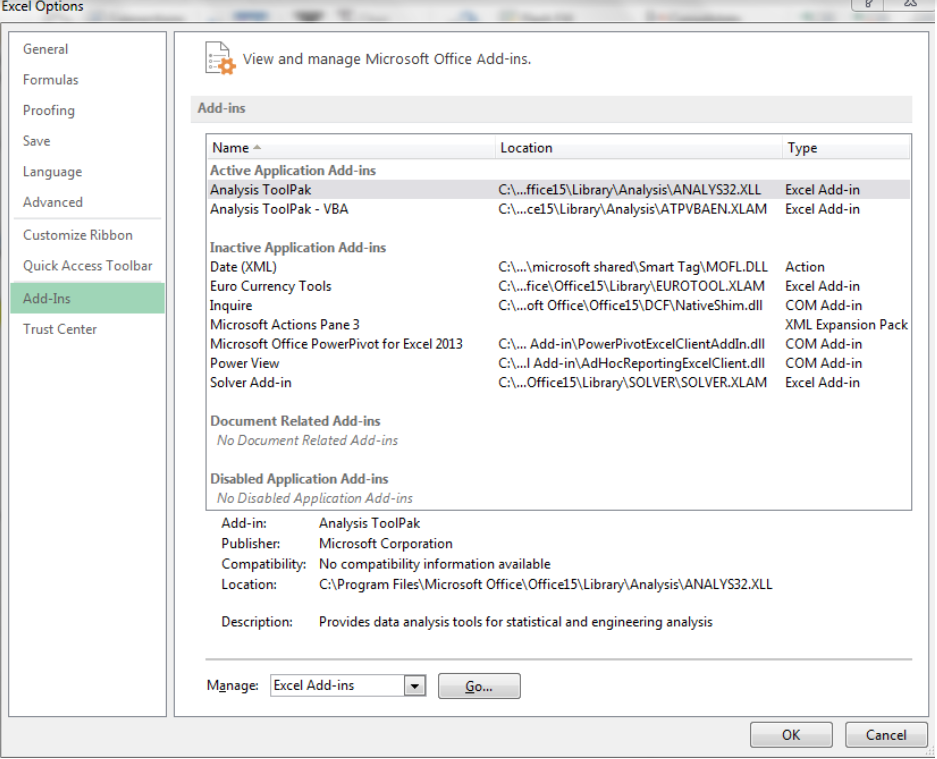Figure 2- Inserting the Data Analysis Option

• Click on Go… at the bottom of figure 2
• Check the Analysis Toolpak box and the Analysis ToolPak- VBA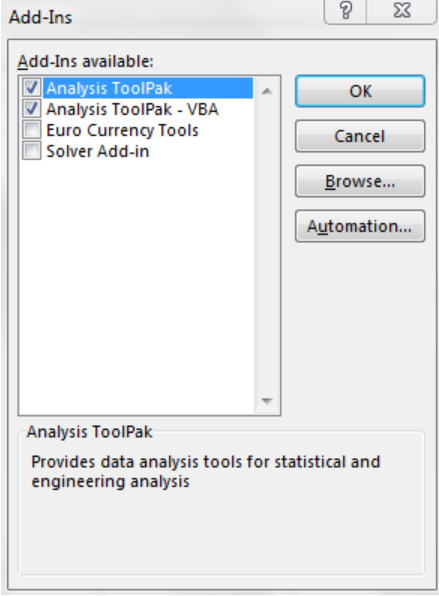Figure 3- Checking the Analysis Toolpak box and Analysis ToolPak- VBA Options

• Press OK.

## Setting up the Data

• We will use the data in Figure 4 to compute the exponential moving average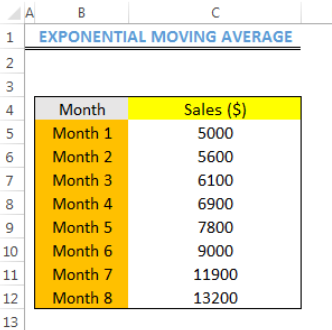Figure 4 – Setting up the Data

## Calculating the Exponential Moving Average

• We will click on Data and then, Data Analysis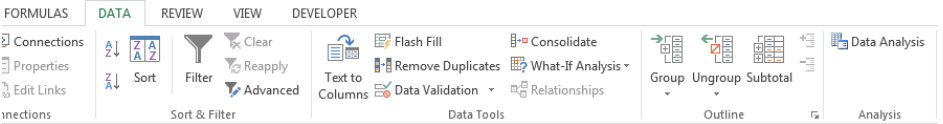Figure 5 – Clicking on Data Analysis

• We will click on Exponential Smoothing and press OK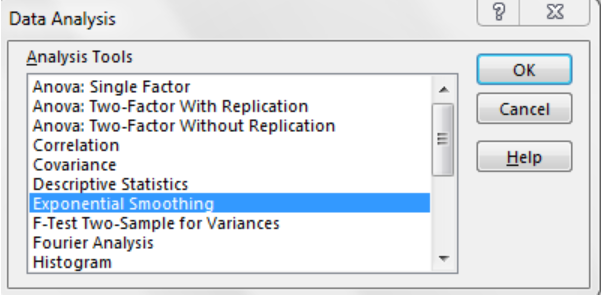Figure 6 – Clicking on Exponential Smoothing

• On the dialog box, we will click on the input range and select Cell C5 to Cell C12. We will input the damping factor as 0.2. We will also insert the output range (Cell D5 to Cell D12) where we want the result to be displayed. We can check the Chart Output and Standard errors boxes if we want them displayed. We will press OK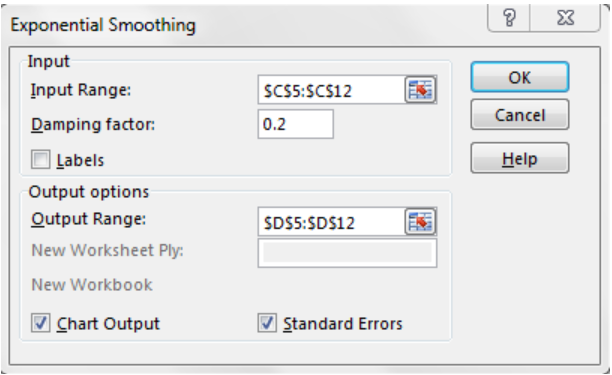Figure 7 – Inserting the Parameters for Exponential Smoothing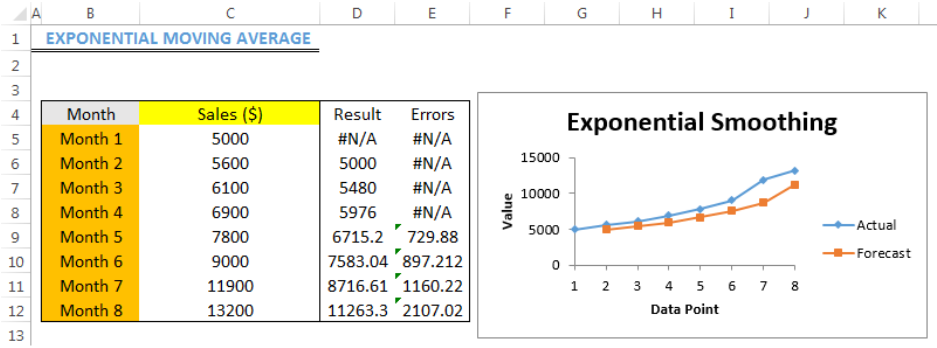Figure 8 – Result of Exponential Moving Average

## Note

• If you have no clue on what you want as the damping factor or smoothing constant, it is best to leave the box empty

## Instant Connection to an Expert through our Excelchat Service

Most of the time, the problem you will need to solve will be more complex than a simple application of a formula or function. If you want to save hours of research and frustration, try our live Excelchat service! Our Excel Experts are available 24/7 to answer any Excel question you may have. We guarantee a connection within 30 seconds and a customized solution within 20 minutes.

Are you still looking for help with the Average function? View our comprehensive round-up of Average function tutorials here.## Subscribe to Excelchat.co